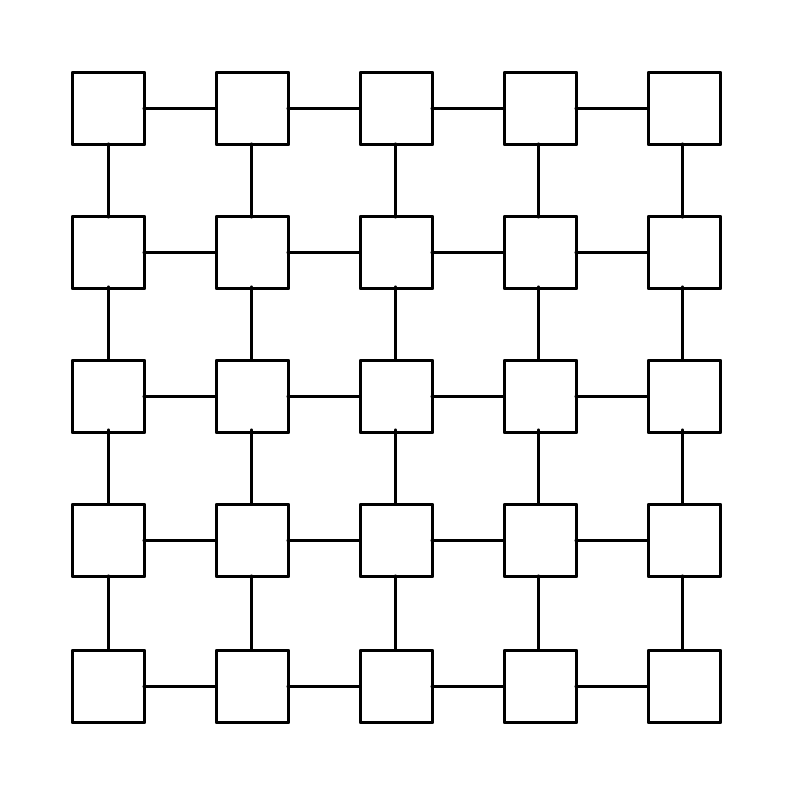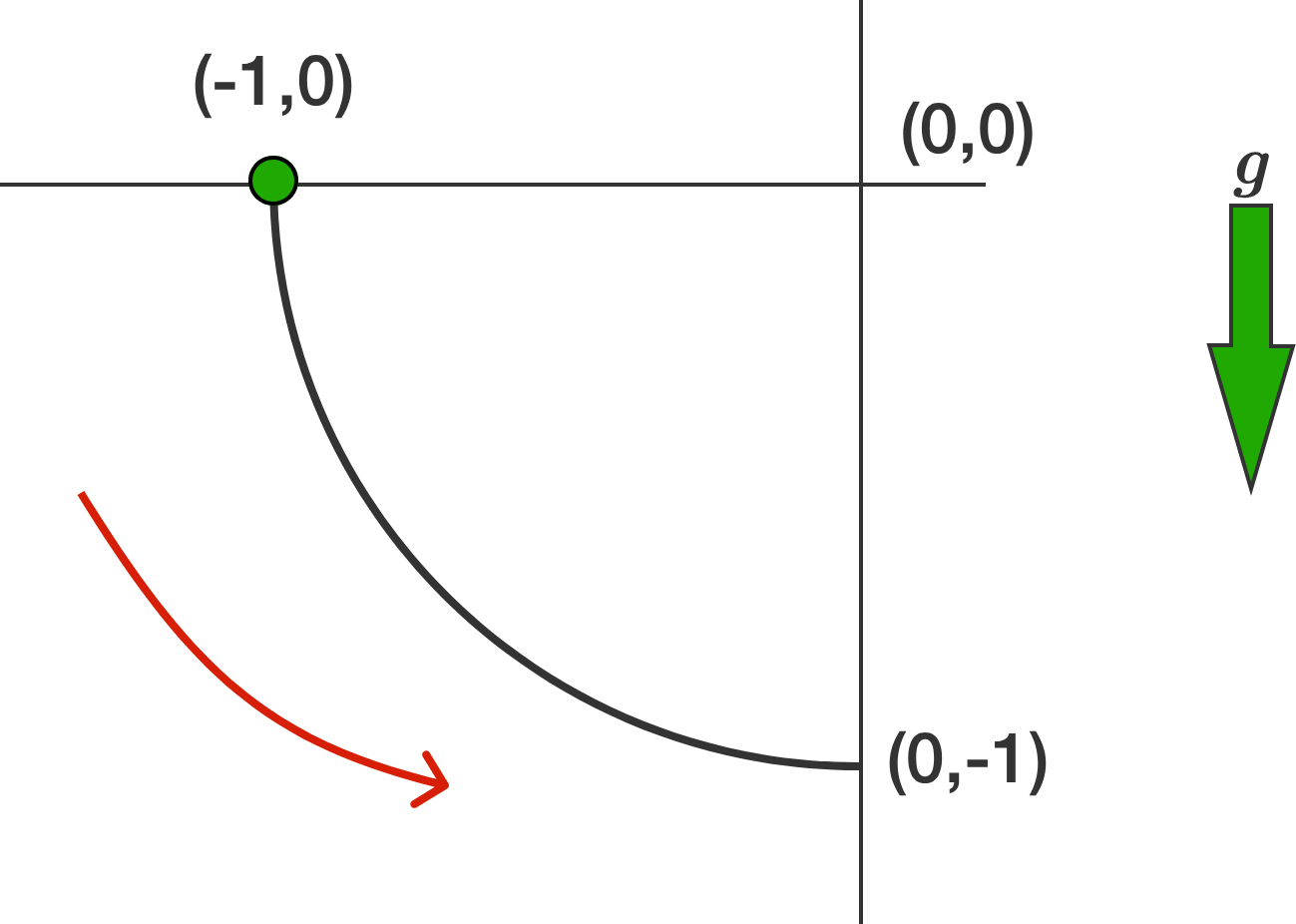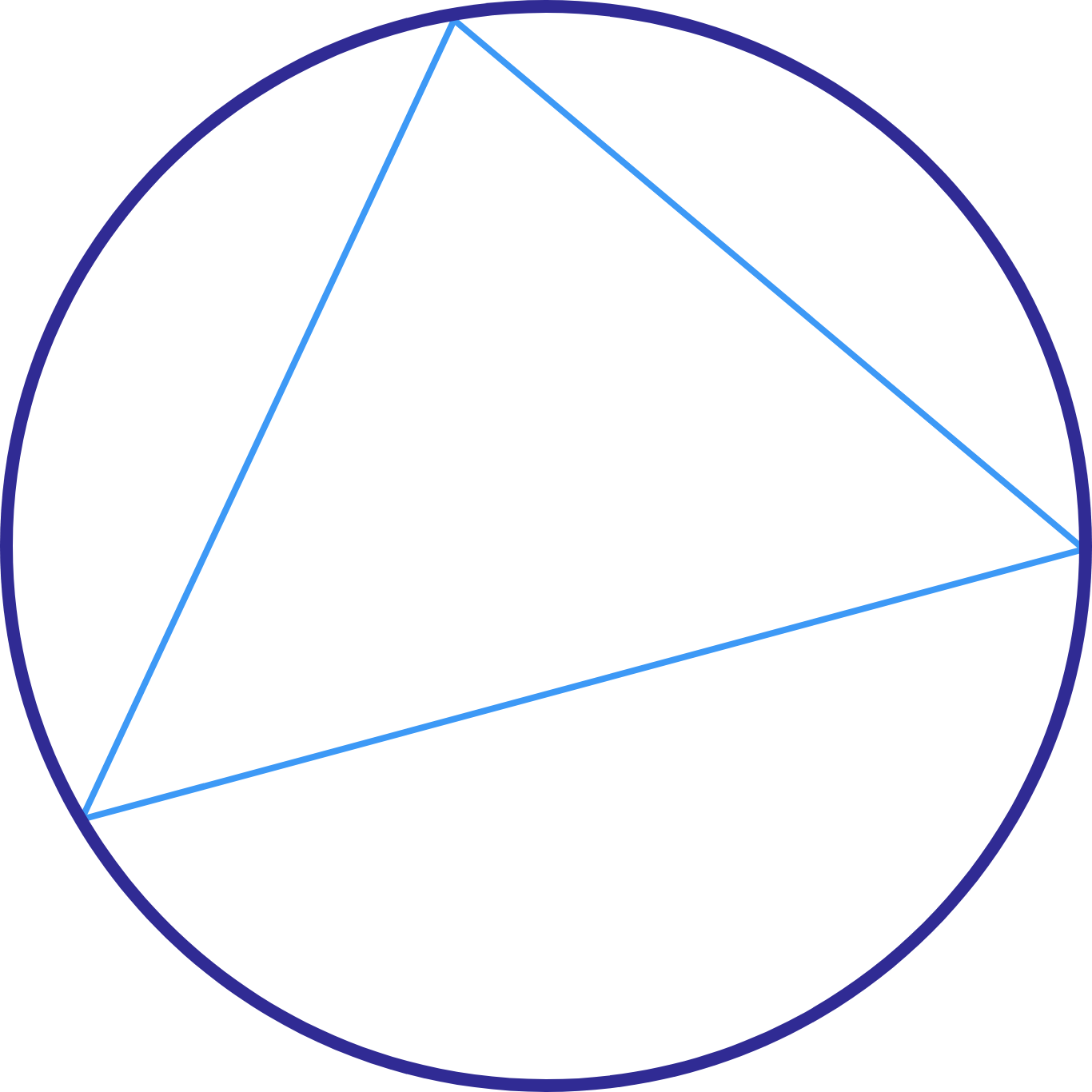# Problems of the Week

Contribute a problem

$X^2 = 2017...$ Find the smallest positive integer $X$ such that the first 4 digits of $X^2$ are 2017.Use 25 distinct positive integers to fill up the grid, such that the integers in any two connected squares have a greatest common divisor that is greater than 1.

What is the smallest possible value of the largest positive integer used?

$f^{f(a)}(b) f^{f(b)}(a) = \big(f(a+b)\big)^2$

Let $f:\mathbb{N} \to \mathbb{N}$ be an injective function such that the above holds true for all $a,b \in \mathbb{N}$. Let $S$ be the sum of all possible values of $f(2017)$. Find $S \bmod{1000}$.


Note: $f^{k} (n)$ means $\underbrace{f\big(f(f(\ldots f(n)\ldots))\big)}_{\text{number of }f \text{'s}\ = \ k}.$A $\SI{1}{\kilo\gram}$ bead slides from rest under the pull of gravity from the point $\left(\SI{-1}{\meter}, \SI{0}{\meter}\right)$ to the point $\left(\SI{0}{\meter}, \SI{-1}{\meter}\right)$ along a wire in the shape of a circle centered on the origin, as shown above.

The wire is rough, and exerts a retarding tangential friction force $F_f = \mu F_\textrm{normal}$ on the bead, with $\mu = \frac{1}{2}.$

Find the speed of the bead $($in $\text{m/s})$ when it arrives at $\left(\SI{0}{\meter},\SI{-1}{\meter}\right).$


Note: $g = \SI[per-mode=symbol]{10}{\meter\per\second\squared}.$

A family of triangles all have the same area which is numerically the same as their perimeters. The greatest circumradius of any of them is exactly twice the least circumradius of any of them. What's the area of a triangle in this family of triangles (to 3 decimal places)?Note: An equilateral triangle with an area of $12 \sqrt{3}$ also has a perimeter of $12 \sqrt{3}$, but this is the only such triangle with this area and perimeter, and hence only one circumradius.

×